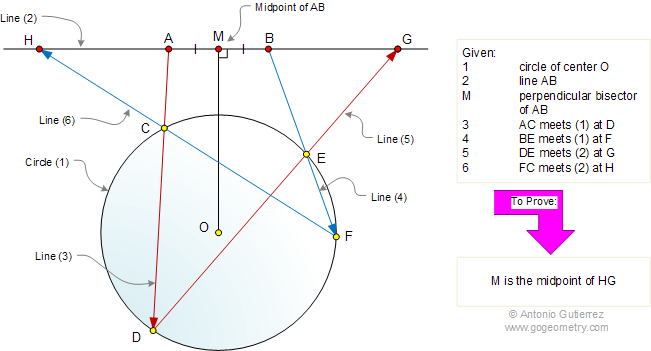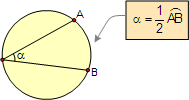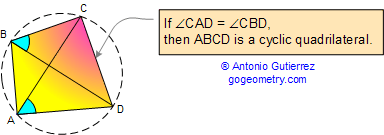Problem 78. Angles in a circle, Perpendicular lines,  Congruence. Level: High School, SAT Prep, College
 Given a circle O, OM is the perpendicular bisector of AB, AC extended meets the circle at D, BE extended meets the circle at F, DE extented and AB extended meet at G, FC extended and BA extended meet at H. Prove that M is the midpoint of HG. View or post a solution.HINTS:

PARALLEL LINES

Proposition. If two lines are parallel, each pair of alternate interior angles are congruent. Also converse.ANGLES IN A CIRCLE

Proposition.  An inscribed angle is measured by one-half its intercepted arc.DIAMETER AND CHORD

Proposition.  A diameter perpendicular to a chord bisects the chord and its arcs..TRIANGLE CONGRUENCE A.S.A. If two angles and the included side of one triangle are congruent to the corresponding parts of another, then the triangles are congruent.CYCLIC QUADRILATERAL is a quadrilateral whose vertices all lie on a single circle.

Proposition 1. Opposite angles of a cyclic (inscribed) quadrilateral are supplementary. Also converse.Proposition 2. A quadrilateral is cyclic if one side subtends congruent angles at the two opposite vertices. Also converse.See: Proposed Problem 77Home | Search | Problems | 71-80 | Perpendicular Bisector | Email | By Antonio Gutierrez